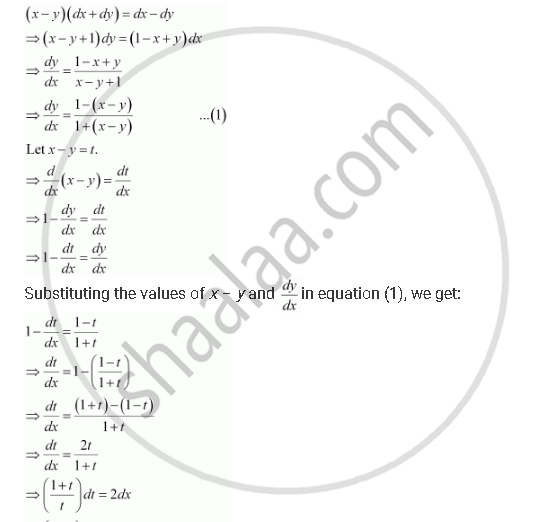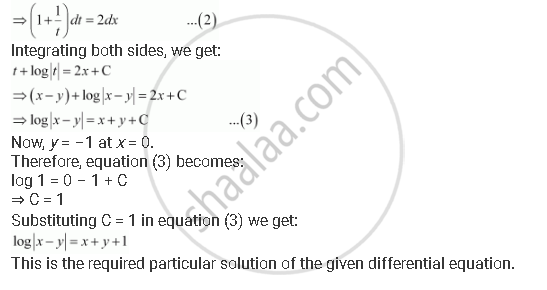Share

# Find a Particular Solution of the Differential Equation (X – Y) (Dx + Dy) = Dx – Dy, Given that Y = –1, When X = 0. (Hint: Put X – Y = T) - CBSE (Science) Class 12 - Mathematics

ConceptFormation of a Differential Equation Whose General Solution is Given

#### Question

Find a particular solution of the differential equation (x – y) (dx + dy) = dx – dy, given that y = –1, when x = 0. (Hint: put x – y = t)

#### SolutionIs there an error in this question or solution?

#### APPEARS IN

NCERT Solution for Mathematics Textbook for Class 12 (2018 to Current)
Chapter 9: Differential Equations
Q: 11 | Page no. 420

#### Video TutorialsVIEW ALL 

Solution Find a Particular Solution of the Differential Equation (X – Y) (Dx + Dy) = Dx – Dy, Given that Y = –1, When X = 0. (Hint: Put X – Y = T) Concept: Formation of a Differential Equation Whose General Solution is Given.
S